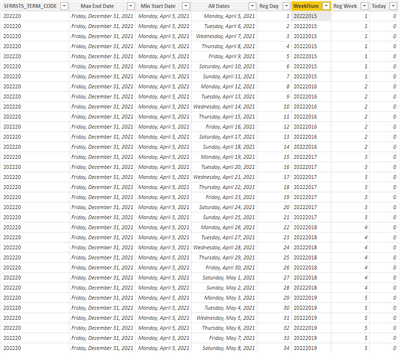cancel
Showing results for
Did you mean:

Fabric is Generally Available. Browse Fabric Presentations. Work towards your Fabric certification with the Cloud Skills Challenge.Frequent Visitor

## Grouping by Week Across Years

Hi,

I have a model of Term Registration Dates that I want to group by registration week, based on the date registration started for each term. I thought I had it working, except now that I brought more data in that crosses into the next calendar year, the calculation I was using falls apart.

Using the earliest and latest dates available for registration for each term, I was able to generate a table that listed all the dates in between. I then used RANX to rank the dates, filtered for each academic term, to get a registration day number. I didn't use this in the week calculation, but I needed the days as well. For the weeks, I had originally pulled the week number and combined it with the term code which is a numeric value as well. This worked fine until January 1 came along on a Sunday.

The idea of this data is to compare year to year registration by day and week. So if I look at Fall 2021 and Fall 2022, they may have had different registration dates, but I can compare day to day and week to week in the respective period.  Following is a very small sample of my data.1 ACCEPTED SOLUTIONSuper User

@GerryL , Assume you have a term start date

//All these column are in a separate date table marked as date table

then you can have columns like

Day of period  = datediff([term Start Date],[date], day) +1

Week of period  = quotient(datediff([term Start Date],[date], day) ,7) +1 //Based on start Date

or

Week of period  =

var _1 = [term Start Date] - weekday([term Start Date],2)+1  //Monday of term start , use 1 for Sunday in weekday

return

quotient(datediff(_1,[date], day) ,7) +1 //Based on start Date

Create rank on term start date

Period Rank = RANKX(all(Period),Period[Term Start Date ],,ASC,Dense)

This Period = CALCULATE(sum('Table'[Qty]), FILTER(ALL(Period),Period[Period Rank]=max(Period[Period Rank])))
Last Period = CALCULATE(sum('Table'[Qty]), FILTER(ALL(Period),Period[Period Rank]=max(Period[Period Rank])-1))

Period Day = CALCULATE(sum('Table'[Qty]), FILTER(ALL('Date'),'Date'[Period Rank]=max('Date'[Period Rank]) && [Period Day] =max([Period Day])))

Last period Day  = CALCULATE(sum('Table'[Qty]), FILTER(ALL('Date'),'Date'[Period Rank]=max('Date'[Period Rank])-1 && [Period Day] =max([Period Day])))

Period Week= CALCULATE(sum('Table'[Qty]), FILTER(ALL('Date'),'Date'[Period Rank]=max('Date'[Period Rank]) && [Week of period] =max([Week of period ])))

Last period Week= CALCULATE(sum('Table'[Qty]), FILTER(ALL('Date'),'Date'[Period Rank]=max('Date'[Period Rank])-1 && [Week of period] =max([Week of period ])))

This video can help to understand

Time Intelligence, DATESMTD, DATESQTD, DATESYTD, Week On Week, Week Till Date, Custom Period on Period,
Custom Period till date: https://youtu.be/aU2aKbnHuWs&t=145s

DAX Calendar - Standard Calendar, Non-Standard Calendar, 4-4-4 Calendar

2 REPLIES 2Super User

@GerryL , Assume you have a term start date

//All these column are in a separate date table marked as date table

then you can have columns like

Day of period  = datediff([term Start Date],[date], day) +1

Week of period  = quotient(datediff([term Start Date],[date], day) ,7) +1 //Based on start Date

or

Week of period  =

var _1 = [term Start Date] - weekday([term Start Date],2)+1  //Monday of term start , use 1 for Sunday in weekday

return

quotient(datediff(_1,[date], day) ,7) +1 //Based on start Date

Create rank on term start date

Period Rank = RANKX(all(Period),Period[Term Start Date ],,ASC,Dense)

This Period = CALCULATE(sum('Table'[Qty]), FILTER(ALL(Period),Period[Period Rank]=max(Period[Period Rank])))
Last Period = CALCULATE(sum('Table'[Qty]), FILTER(ALL(Period),Period[Period Rank]=max(Period[Period Rank])-1))

Period Day = CALCULATE(sum('Table'[Qty]), FILTER(ALL('Date'),'Date'[Period Rank]=max('Date'[Period Rank]) && [Period Day] =max([Period Day])))

Last period Day  = CALCULATE(sum('Table'[Qty]), FILTER(ALL('Date'),'Date'[Period Rank]=max('Date'[Period Rank])-1 && [Period Day] =max([Period Day])))

Period Week= CALCULATE(sum('Table'[Qty]), FILTER(ALL('Date'),'Date'[Period Rank]=max('Date'[Period Rank]) && [Week of period] =max([Week of period ])))

Last period Week= CALCULATE(sum('Table'[Qty]), FILTER(ALL('Date'),'Date'[Period Rank]=max('Date'[Period Rank])-1 && [Week of period] =max([Week of period ])))

This video can help to understand

Time Intelligence, DATESMTD, DATESQTD, DATESYTD, Week On Week, Week Till Date, Custom Period on Period,
Custom Period till date: https://youtu.be/aU2aKbnHuWs&t=145s

DAX Calendar - Standard Calendar, Non-Standard Calendar, 4-4-4 CalendarFrequent Visitor

Thank you! I actually didn't even need to create the rankings for it to work.Announcements#### Power BI Monthly Update - November 2023

Check out the November 2023 Power BI update to learn about new features.#### Fabric Community News unified experience

Read the latest Fabric Community announcements, including updates on Power BI, Synapse, Data Factory and Data Activator.#### The largest Power BI and Fabric virtual conference

130+ sessions, 130+ speakers, Product managers, MVPs, and experts. All about Power BI and Fabric. Attend online or watch the recordings.Top Solution Authors
Top Kudoed Authors
Users online (3,437)